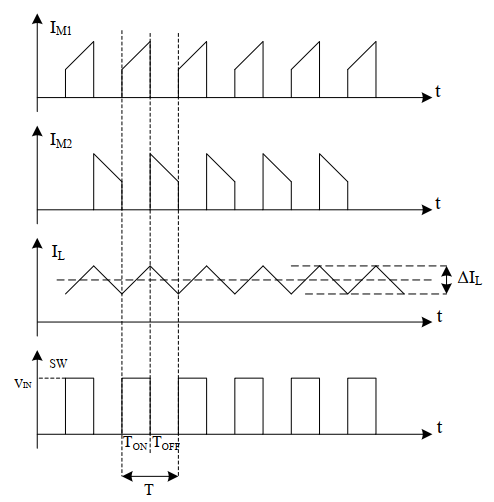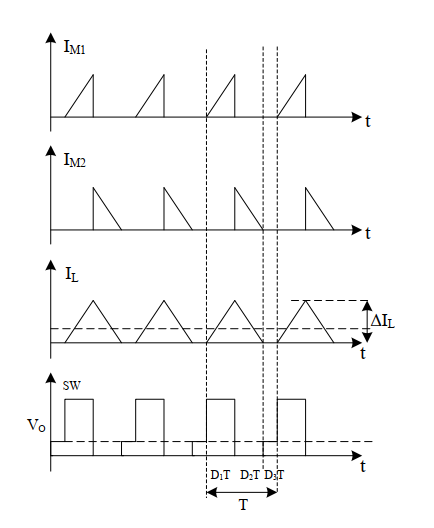# DCDC(BUCK BOOST)基础

buck and boost 电路基础知识，秋招学习笔记

## buck电路### buck的公式计算

$V _{OUT}=V _{IN}*D$

$D=t_{on}*f_s$

$\Delta I_{L} = \frac{V_{OUT} \cdot (1-V_{OUT}/V_{IN})}{L\cdot f_S}$

$L =\frac{V_{OUT}\cdot (1-V_{OUT})/V_{IN}}{\Delta I_{L}\cdot f_{S}}$

$V_{RIPPLE}= \Delta I_{L} \cdot (ESR_{C_{OUT}}+ \frac{1}{8f_sC_{OUT}})$

## boost电路### boost的公式计算

$V_{OUT} = \frac{V_{IN}}{1-D}$

$D=\frac{1-V_{IN}}{V_{OUT}}$

$\Delta I_{L} =\frac{V_{IN}}{L\cdot T_{ON}}= \frac{V_{IN} \cdot (1-V_{IN}/V_{OUT})}{L\cdot f_S}$

$I_{C_{OUT}(RMS)}=I_{OUT(MAX)}\cdot(\sqrt{D/(1-D)})$

## DCDC的损耗

1. 开关管损耗：这是开关电源的主要损耗，主要包括开关损耗、导通损耗。因此应该尽量选择导通电阻比较小的开关管作为开关电源的核心元器件。
2. 电感电容损耗：电感损耗主要包括直流电阻损耗，电容损耗主要包括漏电流损耗。因此应该尽量选择直流电阻较小的电感和漏电流较小的电容元器件。
3. 二极管损耗：主要包括导通损耗和开关损耗。因此应该尽量选择导通压降较小，反向恢复时间较短的二极管，例如肖特基二极管或快恢复二极管等。

## DCDC电路的纹波1. 电感电流纹波通过输出电容的寄生电阻ESR形成的压降
2. 输出电容的充放电
3. 寄生电感引起的电压突变

1. 高频纹波。开关电源的开关管在导通和截止的时候，在电路中就会出现一个与开关上升与下降时间的频率相同或者奇数倍频的噪声，一般为几十 MHz。同样二极管在反向恢复瞬间，其等效电路为电阻电容和电感的串联，会引起谐振，产生的噪声频率也为几十MHz。还有高频变压器的漏感也会产生高频干扰。这些噪声一般叫做高频纹波噪声，幅值通常要比纹波大得多。
2. 开关器件产生的噪声。随着开关的开启和关闭的切换，电感 L中的电流也是在输出电流的有效值上下波动。所以在输出端也会出现一个与开关同频率的纹波。
3. 调节控制环路引起的纹波噪声。实际电路中控制环路要有时间响应，不能做到线性调节，故输出电压瞬间会忽高忽低，甚至有可能造成电源系统的振荡，由此产生了纹波噪声。

1. 在测量输出电压纹波时，要注意如下几点：
2. 保证接地环路尽可能小，建议使用接地环
3. 探头应靠近电容两侧
4. 避免同时使用示波器其他通道测试其他点位的波形
5. 如果只关注开关频率分量纹波，建议打开示波器带宽限制

$V_{pp}=I_{Ripple}\times|Z_{OUT_{eq}}|$

## DCDC 的工作模式

### CCM工作模式

CCM (ContinuousConduction Mode),连续导通模式：在一个开关周期内，电感电流从不会到0。或者说电感从不“复位”，意味着在开关周期内电感磁通从不回到0，功率管闭合时，线圈中还有电流流过。buck变换器在CCM工作模式下的波形

1. 优点
初级峰值电流相对较小，但会叠加较大的直流成分；占空比跟输出的电流大小无关，故适合于负载电流变化较大的场合；对次级输出的电容要求相对较低，有利于降低电容的容量与体积。
2. 缺点
次级整流二极管存在反向恢复的问题，从而引起发热与EMI问题；反馈补偿复杂，存在右半面零点的问题；需要较大的磁芯与较多的初级匝数。

### DCM工作模式

DCM，(Discontinuous Conduction Mode)非连续导通模式：在开关周期内，电感电流总会会到0，意味着电感被适当地“复位”，即功率开关闭合时，电感电流为零。buck变换器在DCM工作模式下的波形

1. 优点
因为初级开关管开通前，次级整流二极管就已经关闭，所以不存在反向恢复的问题；反馈补偿容易，不存在右半面零点的问题，所以负载电流突变引起的瞬态响应更快，动态好，过冲也不会太高。
2. 缺点
所有功率元器件承受的峰值电流都比较大，电流的有效值也大，在一定程度上会影响电路的效率；大的di/dt会带来EMI问题；因为占空比跟输出的电流大小有关，要得到稳定的输出，必定有个最小负载的问题；对次级输出的电容要求也更高，否则会有很大的纹波问题。

https://e2echina.ti.com/blogs_/b/power_house/posts/53234